Journal of Water Resources and Ocean Science
Volume 4, Issue 3, June 2015, Pages: 44-53

Water Velocity, Vorticity and Bed Deviation Modeling for a Reach from Damietta Branch Using K-ε Turbulence Model Solved by Cubic Interpolated Pseudo (CIP) Method

Mohammed Ibrahiem Ibrahiem Mohammed, Mohamed Ahmed Abdel Hady Eid

Irrigation and Hydraulics Department, Faculty of Engineering, Mansoura University, Mansoura, Egypt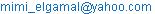(M. I. I. Mohammed)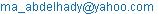(M. A. A. H. Eid)

Mohammed Ibrahiem Ibrahiem Mohammed, Mohamed Ahmed Abdel Hady Eid. Water Velocity, Vorticity and Bed Deviation Modeling for a Reach from Damietta Branch Using K-ε Turbulence Model Solved by Cubic Interpolated Pseudo (CIP) Method. Journal of Water Resources and Ocean Science. Vol. 4, No. 3, 2015, pp. 44-53. doi: 10.11648/j.wros.20150403.11

Abstract: A multi-meander reach with length of 20 km located between km 130.0 and km 150.0 downstream of Delta barrages - Damietta branch was selected and numerically studied. This study aimed to simulate and study numerically the water velocity, vorticity and bed deviation of the curved zones for the reach under study and illustrating the relationship between them. Consequently, the vulnerable zones subjected to maximum velocities were accurately determined. Field data were collected and analyzed for the modeling process. A 3-D model called iRIC (International River Interface Corporative) based on an explicit finite difference method (Abbott-Ionescu scheme) was applied. Therefore, in order to fulfill such objective, standard K-ɛ turbulence model was employed using Cubic Interpolated Pseudo (CIP) method for solving the advection terms. For illustrating obviously the variation of water velocity with vorticity and deviation of bed elevation, two important zones consisted of several meanders were selected, the first zone located from km: 132.00 to km: 137.33 while the second located between km: 137.33 and km: 142.67. Through the modeling process, it was assumed that the sediment particles move in the bed layer zone only. From this study, it was found that for both selected zones, the velocity value was ranged between 0.13 m/sec and 0.24 m/sec, and it could be considered as a small range to make scouring process. It was observed also, that there was a noticeable relationship between water velocity, vorticity and deviation of bed elevation.

Keywords: Flow Characteristics, Modeling, Turbulence Model, Sediment, K-ε model, CIP Method

Contents

1. Introduction

Rivers have been widely considered as an interesting and attractive study subject by engineers and scientists who have been fascinated by the self-formed river geometric shapes and their responses to changes in nature and human interferences. In addition, understanding river behavior and providing flood protection is also essential to environmental enhancement.

Flow in curved river reaches is usually under the influence of centrifugal acceleration, which induces transverse velocity component (helical flow currents) and super elevation in water surface. Although, these curved reaches are sometimes stable, there are general tendency of bank failure and bed scour at the outer bend followed by sedimentation at the inner bend. Therefore, lateral migration of the reach planform occurs, consequently several morphological and navigational problems take place. Due to these dynamic interactions, the transverse velocity profile, shear stress on channel bed, lateral bed slope, sediment size distribution, and energy expenditure will be changed (Grade, 1995).

A meander is a bend in a sinuous watercourse or river. It is formed when the moving water in a stream erodes the outer banks and widens its valley. A stream of any size could be assumed a meandering course, alternately eroding sediment from the outside of a bend and depositing them on the inside. The result is a snaking pattern as the stream meanders back and forth across its down-valley axis. When a meander gets cut off from the main stream, an oxbow lake is formed. (Hickin, 2003).

Stable alluvial river in natural state tends to maintain water conveyance of a specific magnitude by managing its sediment movement and deposition. Variation in local sedimentation, valley slope, geologic properties, and hydrograph influence its geometry as well as the arrangement of the sediment. Therefore, river meandering, lateral migration, deterioration of local navigation depths and flood conveyance are the result of the movement and deposition of bed sediment.

Attia and El-Saied (2004) investigated the statistical nature of river bends along Damietta branch. In this study, three bend types were defined as: free, limited, and forced; which were classified according to the physical and morphological characteristics and degree of freedom to attain the lateral shifting. They concluded that Damietta branch is changing in its planform several times down its course. Also, they summarized meander dimensions of many investigators such as given by Inglis (1938), Leoplod and Wolman (1960, 1964) and Zeller (1967). Based on the analytical regression of the non-linear relationships, there study derived many formulas for Damietta branch concerning the three mentioned types of bends. These formulas linked different parameters of meander geometrical sizes (Ahmed, 2010).

As the combined transport of water and sediment in rivers is a complex process, on-site investigations, evaluation of experience and large scale prototype tests are needed for verifying the results obtained from any mathematical or physical models.

For computation of the bed formation in river bends or near bifurcations, it is important to develop one dimensional models to be two dimensional models. In such models, the two dimensional flow equations in the x and y directions are used.

Three dimensional models need long time and large cost in computations (Wang, 1988; Wang et al., 1989; and Shimizu et al., 1990). In these models the state of turbulence is characterized by turbulence models such as standard k-ɛ, RNG k- ɛ and zero equation models.

Ibrahiem (2015) used iRIC 3-D model for modeling a reach from Damietta branch using upwind scheme for studying numerically the navigation way through this reach. Through his study a comparison between k-ε turbulence model and zero equation turbulence model was illustrated. It was important to illustrate the relationship between flow characteristics and associate between each other. So, in this paper the relationship between water velocity, vorticity and bed deviation was determined accurately through a long reach from Damietta branch of 20 km length using CIP method.

2. Site Description

As Damietta branch is very well concerned by Ministry of Water Resources and Irrigation and Ministry of Transport, Egypt, for passing maximum required discharges as well as to develop such safe navigation waterway, the study reach under consideration was selected. This reach is approximately 20 km long which locates from km 130 to km 150 downstream of Delta barrages, Damietta branch, Fig. (1).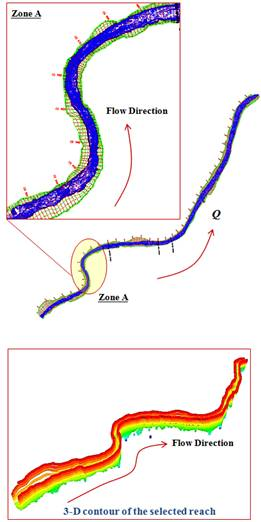Fig. (1). Description of the reach under study.

3. Data Collection

The hydrographic survey of the study reach was carried out by Hydraulics Research Institute "HRI" of the National Water Research Center, Ministry of water resources and Irrigation, Egypt. Using the provided echo-sounder light boat, riverbed bathymetric survey was carried out along the branch following zigzag pathway trans-sections between the two river sides which are roughly spaced at 50 m intervals in stream wise direction. Moreover, in order to cover the study reach area, three longitudinal sections located at the left, middle, and right sides of the river reach were acquired.

Consequently, the provided differential Global Positioning System (GPS) were employed to record each data set point consisting of X and Y positions as well as the flow depth at an interval of one second on the equipped data logger.

Due to the significance of the acquired measurements, differential GPS system was utilized to provide a global accuracy of nearly 1.0 m in the plan direction with a relative depth accuracy of +/-10 cm. While the applied echo-sounder system permits flow depth measurements and consequently determining bed elevation with a relative accuracy of +/- 5 cm. For shallow areas, where the flow depths are less than 0.75 m, another total station system was used which was launched on a light rubber boat (Zodiac). Then, the file of these coordinates (X, Y and Z coordinates) was prepared in the form of (XYZ.tpo) file that would be required for the 3D simulation. This file consisted of 102230 points, each point had X, Y and Z coordinates.

The velocity measurements were carried out at locations of 1.200 km, 9.000 km and 11.839 km from the upstream boundary of the reach under study which is located downstream km 130.

The grab sediment sampler was used to collect 48 bed material samples at different locations to prepare (d50.anc) file used for the 3-D modeling process. The bed sample locations were selected to cover the entire features of the study reach and to represent the difference in the value of the Manning roughness. The samples were analyzed for grain size distribution, according to the relevant specifications, in the Hydraulics Research Institute.

Eight years data between 2005 and 2012 were collected downstream of Delta barrages, for estimating the maximum and minimum discharges from by Hydraulics Research Institute "HRI".

The resulted discharges from analysis of the obtained data could be given as:

The measured discharge (35.50 M. m3/day): is the measured flow discharge during field measurements which equals to 35.50 million m3/day.

The minimum flow discharge (9.90 M. m3/day): is the minimum recorded value downstream of Delta barrages throughout the studied years;

The maximum flow discharge (62.10 M. m3/day): is the maximum recorded value downstream of Delta barrages throughout the investigated years; and

The future discharge (80 M. m3/day): which are stated by the Nile Research Institute as future peak discharges for Damietta branch rehabilitation.

4. Model Set Up

Governing Equations

Momentum equation in X-direction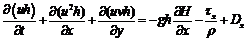(1)

Momentum equation in Y-direction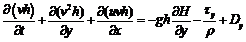(2)

Continuity Equation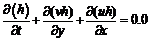(3)

where:

v : velocity in Y-direction;

u : velocity in X-direction;

τx : shear stress at X-direction;

τy : shear stress at Y-direction;

t : time;

h : water depth at any point;

ρ : water density; and

g : gravitational acceleration.

The governing equations were converted from co-orthogonal coordinates (X and Y coordinates) to represent the local stream lines into river coordinates, non-orthogonal coordinate system, (general coordinates or ξ and η coordinates). The non-orthogonal coordinate system allows more precise fitting of the coordinate system to suit arbitrary channel curvature and variable width. More importantly, the more detailed treatment of turbulence and large eddies allow predictions of time-variable behavior even for steady discharges.

Standard K-ε Turbulence Model

Turbulent flow is dissipative, which means that kinetic energy in the small dissipative eddies are transformed into internal energy. The small eddies receive the kinetic energy from slightly larger eddies. The slightly larger eddies receive their energy from even larger eddies and so on. The largest eddies extract their energy from the mean flow. This process of transferred energy from the largest turbulent scales (eddies) to the smallest is called cascade process.

This model is represented by the following equations: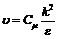(4)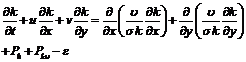(5)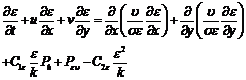(6)

where:

υ : eddy viscosity;

u : water velocity in X-direction;

v : water velocity in Y-direction;

k : turbulence kinetic energy;

ɛ : turbulence dissipation; and

t : time.

and,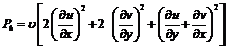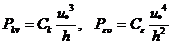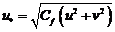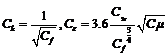where,

 Cμ C1ε C2ε σk σε 0.09 1.44 1.92 1.0 1.30

Sediment Transport Model

In general, it is assumed that the direction of sediment transport is the same as direction of flow and the sediment particles move in the bed layer zone only, Fig. (2).

The general modeling equation is: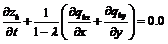where:

Zb : elevation of bed;

t : time;

λ : porosity of sediment mixture;

qbx : sediment rate in X-direction; and

qby : sediment rate in Y-direction.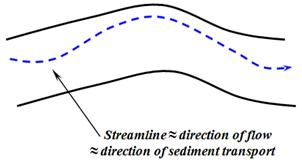Fig. (2). Definition sketch shows the direction of sediment transport.

Watanabe gave the following equations for the sediment transport rates in X and Y directions as (Shimizu, 2012):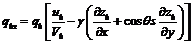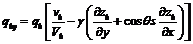according to Hasegwa's formula,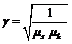where:

µs : static friction factor = 1.0

µk : kinematic friction factor = 0.45

Vegetation Model

For modeling the effect of vegetation, the following equation is included in the model,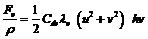where:

Fv : drag force due to vegetation;

C dv : drag coefficient;

λv : vegetation density; and

hv : water depth or vegetation height, Fig. (3).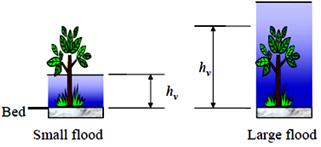Fig. (3). Indication of term hv used in vegetation model.

where,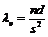s2 is the area of grid cell, n is the number of stems of vegetation in the cell, d is the averaged diameter of each stem, Fig. (4).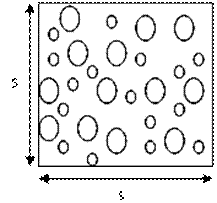Fig. (4). Plan view of vegetation points.

Grid Generation

The three dimensional model called IRIC (International River Interface Corporative), is a model used for supporting the numerical modeling of river morphodynamics using different turbulence models based on an explicit finite difference method (Abbott-Ionescu scheme) with CIP method for solving the advection terms.

The description of grid is given as:

number of streamwise points = 61;

number of cross-stream points = 11;

number of iterations = 25; and

centerline tensions = 10;

standard relaxation coefficient = 0.2.

stream wise increment = 347.63;

cross stream wise increment = 30.00;

number of sub segments = 41;

number of triangles = 191298;

number of vertices = 102230;

number of incircle tests = 804607; and

number of 2D orientation tests = 1072079.

The basic goal of mesh design is creating a representation of the water body that provides an adequate approximation of the true solution of the governing equations.

The stage of network design is finished when the contour of the whole reach can be plotted by the program. The different grid elements for the whole reach could be plotted as given in Fig. (5).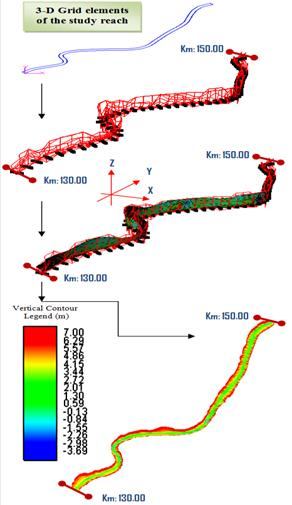Fig. (5). 3-D contour map for the reach under study.

For all runs the following items have to be adjusted:

output time interval = 1 sec;

calculation time step = 0.001 sec (minimum time step);

start time for output = 0.0 sec;

end of time steps calculations= 50 sec; and

start time for bed deformation = 0.0 sec.

The boundary conditions can be specified for all runs as:

-no periodic boundary conditions;

-upstream velocity will be calculated according to uniform flow principles; and

-boundary condition slope will be estimated from geometric data.

-downstream water levels for measured, minimum, maximum and future discharges are 9.00 m, 8.10 m, 9.21 m and 10.00 m, respectively.

According to initial conditions; the initial water surface should be calculated according to the principles of non-uniform The median diameter of bed material (d50) can be entered as a file with the extent of (.anc), and the standard value of critical angle of repose (φ) for bed material is used and equal to 0.3 (Shimizu, 2012).

In this model; both the length and width of the reach are divided into 60 and 10 units, respectively.

5. Modeling Process

In this research, the modeling process was carried out using the measured flow discharge of 410.87 m3/sec.

To illustrate obviously the variation of water velocity with vorticity and deviation of bed elevation, two important zones consisted of several meanders were selected, Fig. (6).

Zone (1): [5.33 km length] lies from km: 132.00 (unit 6) to km: 137.33 (unit 22)

Zone (2) [5.34 km length] locates between km: 137.33 and km: 142.67 (unit 38).

For each zone, three longitudinal sections at 30%Bi (unit 3), 50% Bi (unit 5) and 70%Bi (unit 7) were selected. Where, Bi is the variable width of the reach at any cross section (i).

Fig. (7) shows the contours of water velocity at the two selected zones.

Fig. (8) and Fig. (9) exhibit the water velocity through the three longitudinal sections (at 30% Bi, 50% Bi and 70% Bi) for both zone (1) and zone (2), respectively.

Figs. (10) through (15) illustrate the water velocity, vorticity and bed deviation for the three selected longitudinal sections for zone (1) and zone (2), respectively.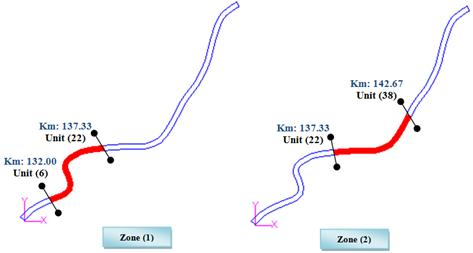Fig. (6). Selected two zones.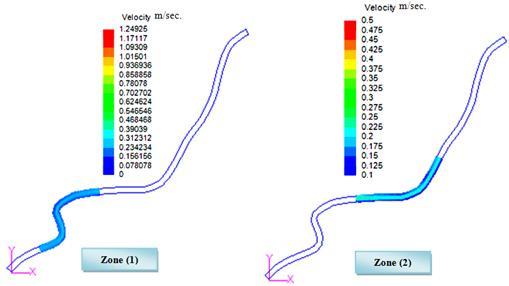Fig. (7). Filled velocity contours for the selected two zones.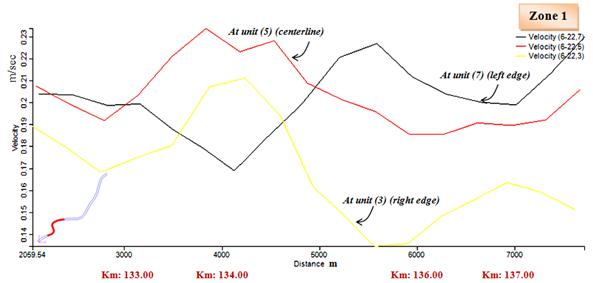Fig. (8). Water velocity for the three selected longitudinal sections at zone (1).Fig. (9). Water velocity for the three selected longitudinal sections at zone (2).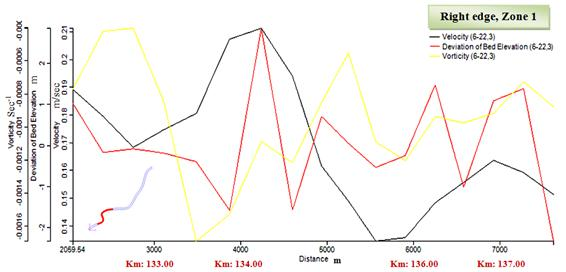Fig. (10). Water velocity, bed deviation and vorticity for zone (1) at longitudinal section (1) [30%Bi, right edge].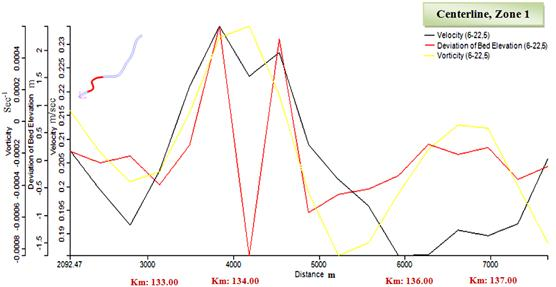Fig. (11). Water velocity, bed deviation and vorticity for zone (1) at longitudinal section (2) [50%Bi, centerline].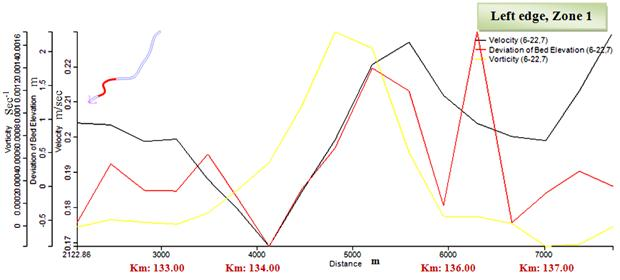Fig. (12). Water velocity, bed deviation and vorticity for zone (1) at longitudinal section (3) [70%Bi, left edge].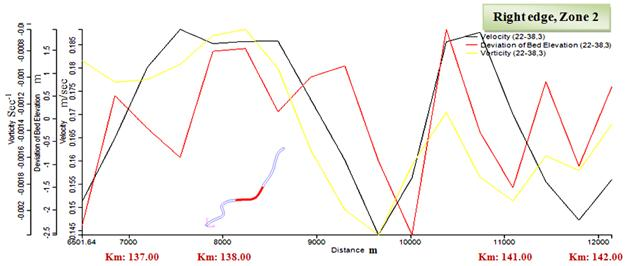Fig. (13). Water velocity, bed deviation and vorticity for zone (2) at longitudinal section (1) [30%Bi, right edge].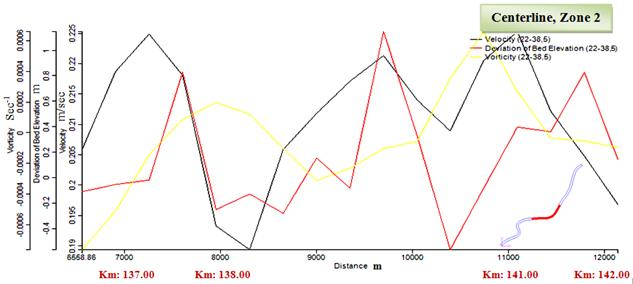Fig. (14). Water velocity, bed deviation and vorticity for zone (2) at longitudinal section (2) [50%Bi, centerline].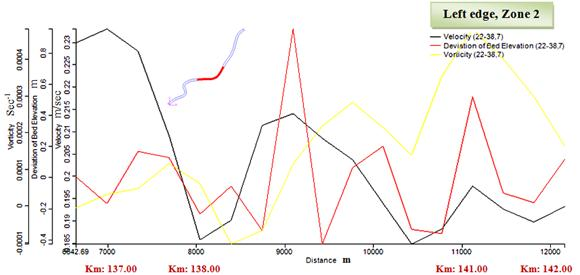Fig. (15). Water velocity, bed deviation and vorticity for zone (2) at longitudinal section (3) [70%Bi, left edge].

From Figs. (8) and (9), it was observed that:-

- For zone (1), the water velocity ranged from 0.13 m/sec to 0.21 m/sec at right edge, from 0.17 m/sec to 0.23 m/sec for left edge and from 0.19 m/sec to 0.24 m/sec at the centerline.

- For zone (2), the water velocity ranged from 0.14 m/sec to 0.19 m/sec at right edge, from 0.19 m/sec to 0.23 m/sec for left edge and from 0.19 m/sec to 0.23 m/sec at the centerline.

- For zone (1), the maximum value of velocity at the right edge, left edge and centerline was found at km: 134.5, km: 135.8 and km: 133.85, respectively.

- For zone (2), the maximum value of velocity at the right edge, left edge and centerline was found at km: 137.5, km: 137.2 and km: 141.3, respectively.

It was noticed from these figures also, that the water velocity at the left edge and centerline was higher than the corresponding one at the right edge of the reach for both zones. This means that the effect of centrifugal force for bends here is very small and its effect could be neglected. It was also found that for both zones the values of velocities were ranged between 0.13 m/sec and 0.24 m/sec, and this range could be considered small to make scouring process.

It is important also to explain that these velocity values could arrive to the sedimentation range and may cause a serious danger for formation islands in this reach.

From analysis of Figs. (10) through (15), it was showed that there was a noticeable relationship between water velocity and vorticity for both zones, because when the water velocity increased the corresponding vorticity decreased and the corresponding bed deviation increased.

This action occurs when the X and Y components of velocity increases in the longitudinal direction and then, the corresponding vorticity of water in the vertical direction decreases and the deviation of bed elevation could increase.

Also, it was determined that the values of vorticity at the center line of the reach ranged between - 0.0008 sec-1 and + 0.0004 sec-1 for zone (1) and ranged from -0.0006 sec-1 to + 0.0006 sec-1 for zone (2), while the vorticity values at the right edge were higher than the corresponding ones at the left edge.

The vorticity values at the centerline of the reach were less than the corresponding ones for both right and left edges.

Fig. (16) show the resulted deviation of bed elevation for the whole reach starting from km: 130.00 to km: 150.00. It is resulted from this figure that there was a noticeable sedimentation process covered the most of the reach.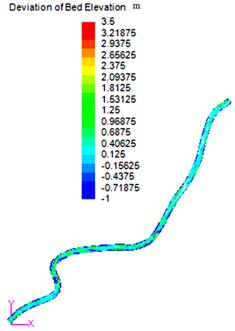Fig. (16). Filled contours for resulted bed deviation.

6. Conclusions

A multi-meandering reach with length of around 20 km located between km 130 and km 150 downstream of Delta barrages - Damietta branch was selected and numerically studied. The aims of this study were simulating and modeling numerically the water velocity, vorticity and bed deviation for the curved zones of the reach under study and then, to determine accurately the vulnerable zones subjected to maximum velocities. Field data were collected and analyzed for the modeling process. A 3-D model called iRIC (International River Interface Corporative) based on an explicit finite difference method (Abbott-Ionescu scheme) was used. A standard K-ɛ turbulence model was employed using Cubic Interpolated Pseudo (CIP) method for solving the advection terms. The effect of vegetation and interactions between sediment motion and vegetation were added in the modeling process.

From this study, it was observed that the water velocity at the left edge and centerline was higher than the corresponding one at the right edge of the reach for the selected curved zones. This means that the effect of centrifugal force for bends here is very small and its effect could be neglected.

It was also found that for both selected curves, the values of velocities were ranged between 0.13 m/sec and 0.24 m/sec, and this range could be considered small to make scouring process, and then it could arrive to the sedimentation range and cause a serious danger for formation islands in this reach.

It was showed that there was a noticeable relationship between water velocity, vorticity and deviation of bed elevation for curved zones.

Acknowledgments

Grateful thanks for all staff of Hydraulics Research Institute (HRI), National Water Research Center, for their help in obtaining data.

References

1. Ahmed A. F. (2010). "Improving navigation in river bends by bottom vanes", Scientific Bull., Faculty of Engineering, Ain Shams University, ISSN 1110-1385, Vol. 32, No. 1.
2. Attia K. M. and El-Saied N.A. (2004). "Plan form geometry of river meander at Damietta branch", Scientific Bulletin, Faculty of Engineering, Ain Shams University, ISSN 1110-1385, Vol. 39, No. 1, pp.359-379.
3. Grade R. J. (1995). "History of fluvial hydraulics", New Age Publishers. p. 14.and p.19, ISBN 812240815X. OCLC 34628134.
4. Hickin J.S. (2003). "Meandering channels", International Journal of Recent Trends in Engineering, India, Vol. 1, No. 6, pp. 430–434.
5. Ibrahiem, M.I., Zidan, A.A., El-Alfy, K.S. and Abdalla, M. G. (2015). "Numerical modeling of flow conditions and bed deviations of a reach from the river Nile", PhD thesis, Irrigation and Hydraulics Dept., El-Mansoura University.
6. Inglis C.D. (1938). "Relationship between meander belts", Central Irrigation Hydrodynamic, India, Tech. Note No. 12.
7. Leopold L. B. and Wolman M. G. (1960). "River meanders", Geological Society, Bull., Vol. 71, pp. 769-794.
8. Leopold L. B., Wolman M. G., and Miller J. P. (1964). "Fluvial processes in geomorphology", W. H. Freeman, San Francisco, California.
9. Shimizu Y.R., Itakura T.G., and Yamaguchi H.K. (1990). "Three dimensional computation of flow and bed deformation", Journal of Hydraulics, ASCE, Vol.116, No. 9, pp. 1090-1108.
10. Shimizu Y.R. (2012). Lecture Notes, "3-D river hydraulics modeling", Hokkaido University, Japan.
11. Wang S.S. (1988). "Three dimensional models for fluvial hydraulic simulation", Proceeding of the International Conference on Fluvial Hydraulics, Budapest, Hungary, 88-30.
12. Wang S.S., Combs P.X., and Hu K.K. (1989). "New developments in modeling 3D sedimentation phenomena", New Orleans, Louisiana, Vol.14, No.18, pp. 33-38
13. Zeller J.D. (1967). "Meander channels in Switzerland", Cranfield University Report, Britannia.

 Contents 1. 2. 3. 4. 5. 6.
Article ToolsAbstractPDF(789K)